Leena, a clever lady lived in a house along with his 2 children. One day, a lady beggar went to Leena's house. Leena, the clever lady asked the beggar how much she get a day. "10 rupees" the lady beggar replied.

Leena felt sorry and gave her a job with a salary of 16 rupees/day along with free food. Along with that, she put forward a condition too... The condition was that, If the beggar is in leave, she has to give 20 rupees/day to Leena.

Beggar accepted the condition and joined for work. After 36 days Leena checked the attendance register for payment, but there was no amount payable. If so, how many days she went for job in that 36 days?

1.2.3.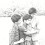4.she work for 20 days so amount is ( 16*20 = 320 )
and took leave for 16 days ( 20*16 = 320)
total amt 320 - 320 = 0

5.6.7.let no. of days she worked is 'x'
then,16(x)-20(36-x)=0
on solving x=20

8.20 days = 20*16=320
16 days = 16*20=320

9.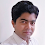Begger worked 20 days...

10.She Worked for 20 days, 16 days on Leave

11.12.she had 20 days of work

13.She worked 20 days and was away for 16 days.

14.15.Musthaq
She worked 20 days as per condition, 20*16 = 320
16*20 = 320, no amount payable...

16.its 20 days 16X-20(36-X)=0 =>X=20 Days.

17.18.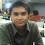u all clever... its 20 days.

19.she worked 7 and half days

20.21.22.23.lol the fact that I actually figured this out and seen the comments agree with me is a great feeling. :P She worked 20 days (and if though it wasn't part of the question) she missed 16 days.

1.Oops! I meant even though, not if though lol.

24.lol the fact that I actually figured this out and seen the comments agree with me is a great feeling. :P She worked 20 days (and if though it wasn't part of the question) she missed 16 days.

25.She worked for 20 days and she did not go to work for 16 days

26.Love the first sentence - Leena, a clever LADY lived in a house along with HIS 2 children.

1.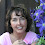My thought exactly. When I see mistakes like that I quit reading..

2.This comment has been removed by the author.

3.There are so many grammatical errors in this question that it's hard to even understand what is being asked!

27.28.I agree with everyone else she worked 20 days

29.That would be correct
20 days

30.That would be correct
20 days

31.That would be correct
20 days

32.20 days *16 =320 - 16 days*20 =320 = 0

33.34.This comment has been removed by the author.

35.0=16*x - 20*(36-x)
0=16*x - 720 + 20*x
720=36x
20=x
verify:
16*20-20*16=0

Answer is that she worked for 20 days.

36.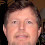This is 8th grade math. X is the number of days worked. 36 - X is the number of days of leave taken. So, 16(X) = 20 (36-X). This yields 36X=720, this X=20 days worked and (36 - x)= 16 days leave taken.

37.This is 8th grade math. X is the number of days worked. 36 - X is the number of days of leave taken. So, 16(X) = 20 (36-X). This yields 36X=720, this X=20 days worked and (36 - x)= 16 days leave taken.

38.This is 8th grade math. X is the number of days worked. 36 - X is the number of days of leave taken. So, 16(X) = 20 (36-X). This yields 36X=720, this X=20 days worked and (36 - x)= 16 days leave taken.

39.This is 8th grade math. X is the number of days worked. 36 - X is the number of days of leave taken. So, 16(X) = 20 (36-X). This yields 36X=720, this X=20 days worked and (36 - x)= 16 days leave taken.

40."Leena, a clever lady... lived with his children." Does anyone on this website have English as their first language?

1.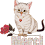her children not his

2.i'm with you notsocrazy!!

41.20 working days

42.43.44.45.46.This comment has been removed by the author.

47.Earn = Pr(Dp)-Ar(36days-Dp)
0 = [16(DP)]-[20(36-Dp)]
0 = 16Dp-720-20Dp
0 = 36Dp-720
720 = 36Dp
Dp = 720/36 = 20days duty; 14days leave

48.there is no way to answer this correctly because the person who wrote this brain teaser doesn't know how to speak proper english and doesn't know if leena is a man or a woman!!

49.50.24 days went on duty.,and 12 days leave..that is 24 days =Rs.240(24*10)and 12 days =Rs. 240(12*20)...acording to the condition Rs.240-Rs.240=0

51.52.16X=20(36-X)
X=20 days